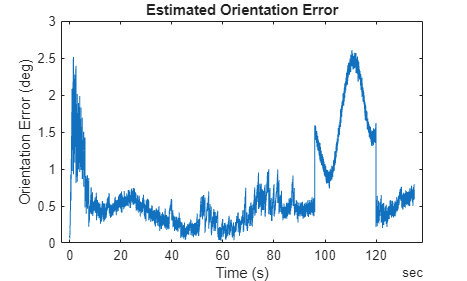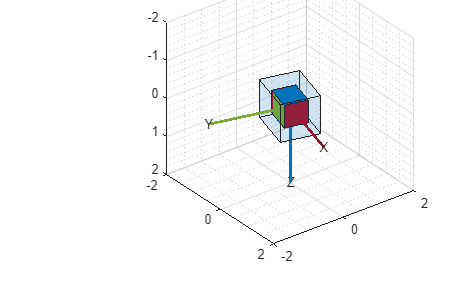# Estimate Orientation Using GPS-Derived Yaw Measurements

This example shows how to define and use a custom sensor model for the `insEKF` object along with built-in sensor models. Using a custom yaw angle sensor, an accelerometer, and a gyroscope, this example uses the `insEKF` object to determine the orientation of a vehicle. You use the velocity from a GPS receiver to compute the yaw of the vehicle. Following a similar approach as shown in this example, you can develop custom sensor models for your own sensor fusion applications.

This example requires either the Sensor Fusion and Tracking Toolbox or the Navigation Toolbox. This example also optionally uses MATLAB Coder to accelerate filter tuning.

### Problem Description

You are trying to estimate the orientation of a vehicle while it is moving. The only sensors available are an accelerometer, a gyroscope, and a GPS receiver that outputs a velocity estimate. You cannot use a magnetometer because there is a large amount of magnetic interference on the vehicle.

#### Approach

There are several tools available in the toolbox to determine orientation including

• `ecompass`

• `imufilter`

• `ahrsfilter`

• `complementaryFilter`

However, these filters require some combination of an accelerometer, a gyroscope, and/or a magnetometer. If you need to determine the absolute orientation (relative to True North) and do not have a magnetometer available, then none of these filters is ideal. The imufilter and complementaryFilter objects fuse accelerometer and gyroscope data, but they only provide a relative orientation. Furthermore, while these filters can estimate and compensate for the gyroscope bias, there is no additional sensor to help track the gyroscope bias for the yaw angle, which can yield a suboptimal estimate. Typically, a magnetometer is used to accomplish this. However, as mentioned, magnetometers cannot be used in situations with large, highly varying magnetic disturbances. (Note that the `ahrsfilter` object can handle mild magnetic disturbances over a short period of time).

In this example, to get the absolute orientation you use the GPS velocity estimate to determine the yaw angle. This yaw angle estimate can serve the same purpose as a magnetometer without the magnetic disturbance issues. You will build a custom sensor in the `insEKF` framework to model this GPS-based raw sensor.

#### Trajectory

First, you create a ground truth trajectory and simulate the sensor by using the `exampleHelperMakeTrajectory` and `exampleHelperMakeIMUGPSData` functions attached with this example

```groundTruth = exampleHelperMakeTrajectory; originalSensorData = exampleHelperMakeIMUGPSData(groundTruth);```

Retuned as a timetable, the original sensor data includes the accelerometer, gyroscope, and GPS velocity data. Transform the GPS velocity data into a yaw angle estimate.

`velyaw = atan2(originalSensorData.GPSVelocity(:,2), originalSensorData.GPSVelocity(:,1)); `

The above results assume nonholonomic constraints. That is, they assume the vehicle is pointing in the direction of motion. This assumption is generally true some vehicles, such as a car, but not true for some other vehicles, such as a quadcopter.

#### Create Synthetic Yaw Angle Sensor Data

Yaw angle is difficult to work with because the yaw angle wraps at $\pi$ and $-\pi \text{\hspace{0.17em}}$. The angle jumping at the wrapping bound could cause divergence of the extended Kalman filter. To avoid this problem, convert the yaw angle to a quaternion.

```velyaw(:,2) = 0; % set pitch and roll to 0 velyaw(:,3) = 0; qyaw = quaternion(velyaw, 'euler', 'ZYX', 'frame');```

A common convention is to force the quaternion to have a positive real part

```isNegQuat = parts(qyaw) < 0; % Find quaternions with a non-negative real part qyaw(isNegQuat) = -qyaw(isNegQuat); % Invert the negative quaternions.```

Note that when the insEKF object tracks a 4-element `Orientation` state as in this example, it assumes the `Orientation` state is a quaternion and enforces the quaternion to be normalized and have a positive real part. You can disable this rule by calling the state something else, like "Attitude".

Now you can build the timetable of the sensor data to be fused.

```sensorData = removevars(originalSensorData, 'GPSVelocity'); sensorData = addvars(sensorData,compact(qyaw),'NewVariableNames','YawSensor');```

#### Create an `insEKF `Sensor for Fusing Yaw Angle

To fuse the yaw angle quaternion, you customize a sensor model and use it along with the `insAccelerometer` and `insGyroscope` objects in the `insEKF` object. To customize the sensor model, you create a class that inherits from the` positioning.INSSensorModel `interface class and implement the measurement method. Name the class `exampleHelperYawSensor`

```classdef exampleHelperYawSensor < positioning.INSSensorModel %EXAMPLEHELPERYAWSENSOR Yaw angle as quaternion sensor % This class is for internal use only. It may be removed in the future. % Copyright 2021 The MathWorks, Inc. methods function z = measurement(~, filt) % Return an estimate of the measurement based on the % state vector of the filter saved in filt.State. % % The measurement is just the quaternion converted from the yaw % of the orientation estimate, assuming roll=0 and pitch=0. q = quaternion(stateparts(filt, "Orientation")); eul = euler(q, 'ZYX', 'frame'); yawquat = quaternion([eul(1) 0 0], 'euler', 'ZYX', 'frame'); % Enforce a positive quaternion convention if parts(yawquat) < 0 yawquat = -yawquat; end % Return a compacted quaternion z = compact(yawquat); end end end ```

Now use a `exampleHelperYawSensor` object alongside an `insAccelerometer` object and an `insGyroscope` object to construct the `insEKF` object.

```opts = insOptions(SensorNamesSource='property', SensorNames={'Accelerometer', 'Gyroscope', 'YawSensor'}); filt = insEKF(insAccelerometer, insGyroscope, exampleHelperYawSensor, insMotionOrientation, opts);```

Initialize the filter using the `stateparts` and `statecovparts` object functions.

```stateparts(filt, 'Orientation', sensorData.YawSensor(1,:)); statecovparts(filt,'Accelerometer_Bias', 1e-3); statecovparts(filt,'Gyroscope_Bias', 1e-3);```

#### Filter Tuning

You can use the `tune` object function to find the optimal noise parameters for the `insEKF` object. You can directly call the `tune` object function, or you can use a MEX-accelerated cost function with MATLAB Coder.

```% Trim ground truth to just contain the Orientation for tuning. trimmedGroundTruth = timetable(groundTruth.Orientation, ... SampleRate=groundTruth.Properties.SampleRate, ... VariableNames={'Orientation'}); % Use MATLAB Coder to accelerate tuning by MEXing the cost function. % To run the MATLAB Coder accelerated path, prior to running the example, % type: % exampleHelperUseAcceleratedTuning(true); % To avoid using MATLAB Coder, prior to the example, type: % exampleHelperUseAcceleratedTuning(false); % By default, the example will not tune the filter live and will not use % MATLAB Coder. % Select the accelerated tuning option. acceleratedTuning = exampleHelperUseAcceleratedTuning(); if acceleratedTuning createTunerCostTemplate(filt); % A new cost function in the editor % Save and close the file doc = matlab.desktop.editor.getActive; doc.saveAs(fullfile(pwd, 'tunercost.m')); doc.close; % Find the first parameter for codegen p = tunerCostFcnParam(filt); %#ok<NASGU> % Now generate a mex file codegen tunercost.m -args {p, sensorData, trimmedGroundTruth}; % Use the Custom Cost Function and run the tuner for 20 iterations tunerIters = 20; cfg = tunerconfig(filt, MaxIterations=tunerIters, ... Cost='custom', CustomCostFcn=@tunercost_mex, ... StepForward=1.5, ... ObjectiveLimit=0.0001, ... FunctionTolerance=1e-6, ... Display='none'); mnoise = tunernoise(filt); tunedmn = tune(filt, mnoise, sensorData, trimmedGroundTruth, cfg); else % Use optimal parameters obtained separately. tunedmn = struct(... 'AccelerometerNoise', 0.7786515625000000, ... 'GyroscopeNoise', 167.8674323338237, ... 'YawSensorNoise', 1.003122320344434); adp = diag([... 1.265000000000000; 1.181989791398048; 0.735171900658607; 0.765000000000000; 0.026248409763699; 0.154586330266264; 31.823154516336434; 0.000546245218270; 5.517012554348883; 0.809085937500000; 0.139035477206961; 41.340145917279159; 0.592875000000000]); filt.AdditiveProcessNoise = adp; end```

#### Fuse Data and Compare to Ground Truth

Batch fuse the data with the `estimateStates` object function.

`est = estimateStates(filt, sensorData, tunedmn)`
```est=13500×5 timetable Time Orientation AngularVelocity Accelerometer_Bias Gyroscope_Bias StateCovariance ________ ______________ ______________________________________ _________________________________________ ______________________________________ _______________ 0 sec 1x1 quaternion 0.00029964 0.0003257 0.00033221 3.1017e-08 -3.4836e-24 -1.2416e-09 2.9964e-07 3.257e-07 3.3221e-07 {13x13 double} 0.01 sec 1x1 quaternion 0.00058811 0.0010514 0.00077335 -1.7319e-10 -1.9136e-05 1.5274e-06 1.0727e-06 0.00013317 2.6591e-06 {13x13 double} 0.02 sec 1x1 quaternion 0.0010153 0.0011293 0.0012812 1.1835e-08 5.0402e-05 4.4661e-06 2.2303e-06 0.0003694 6.6701e-06 {13x13 double} 0.03 sec 1x1 quaternion 0.0013953 0.0014522 0.0019019 1.0275e-08 9.5573e-05 2.0662e-06 3.7974e-06 0.00073969 1.2675e-05 {13x13 double} 0.04 sec 1x1 quaternion 0.0017109 0.0015025 0.0025803 2.3834e-08 0.00010594 6.3287e-06 5.7708e-06 0.0012283 2.0107e-05 {13x13 double} 0.05 sec 1x1 quaternion 0.00205 0.0019622 0.0033511 1.4978e-08 0.00012105 5.2769e-06 8.2924e-06 0.0018652 2.9309e-05 {13x13 double} 0.06 sec 1x1 quaternion 0.0020261 0.0023534 0.0041042 8.8652e-09 -6.6667e-05 1.5446e-06 1.1182e-05 0.0025908 3.8877e-05 {13x13 double} 0.07 sec 1x1 quaternion 0.0026568 0.0026599 0.0050163 8.3994e-09 0.00012693 4.528e-06 1.4392e-05 0.0034661 5.0998e-05 {13x13 double} 0.08 sec 1x1 quaternion 0.0027766 0.0029837 0.0058779 2.101e-09 3.3626e-05 1.1473e-05 1.7889e-05 0.0042033 6.2921e-05 {13x13 double} 0.09 sec 1x1 quaternion 0.0032068 0.0030261 0.0068784 1.3912e-08 0.00011826 1.7097e-05 2.1816e-05 0.0051831 7.7173e-05 {13x13 double} 0.1 sec 1x1 quaternion 0.0031981 0.0032051 0.007876 1.643e-08 -7.5163e-05 1.2083e-05 2.6806e-05 0.0061659 9.1711e-05 {13x13 double} 0.11 sec 1x1 quaternion 0.0036603 0.0032823 0.008879 2.4021e-08 3.2796e-05 1.2375e-05 3.1437e-05 0.00716 0.00010671 {13x13 double} 0.12 sec 1x1 quaternion 0.003734 0.0034359 0.0099138 2.7443e-08 -9.4583e-05 8.6765e-06 3.6787e-05 0.0083283 0.0001224 {13x13 double} 0.13 sec 1x1 quaternion 0.0039583 0.0036587 0.011055 2.6296e-08 -0.00012251 3.6091e-06 4.2167e-05 0.0095194 0.00014002 {13x13 double} 0.14 sec 1x1 quaternion 0.0040944 0.0040022 0.012244 1.7306e-08 -0.0002173 2.531e-06 4.8518e-05 0.01074 0.00015864 {13x13 double} 0.15 sec 1x1 quaternion 0.0044477 0.0040462 0.013476 2.4033e-08 -0.00015984 8.1767e-06 5.4321e-05 0.011837 0.00017817 {13x13 double} ⋮ ```

Compare the estimated orientation to ground truth orientation. Plot the quaternion distance between the estimated and true orientations.

```% Convert compacted quaternions to regular quaternions estOrient = quaternion(est.Orientation); d = rad2deg(dist(estOrient, groundTruth.Orientation)); plot(groundTruth.Time, d); xlabel('Time (s)') ylabel('Orientation Error (deg)'); title('Estimated Orientation Error');````snapnow;`

From the results, the estimated orientation error is low overall. There is a small bump in the error around 100 seconds, which is likely due to a slight inflection in the ground truth yaw angle at the time. But overall, this is a good orientation estimate result for many applications. You can visualize the estimated orientation using the `poseplot` function.

```p = poseplot(estOrient(1)); for ii=2:numel(estOrient) p.Orientation = estOrient(ii); drawnow limitrate; end```### Conclusion

In this example, you learned how to customize a sensor and add it to the `insEKF` framework. Custom sensors can integrate with the built-in sensors like `insAccelerometer` and `insGyroscope`. You also learned how to use the tune object function to find optimal noise parameters and improve the filtering results.